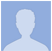## geoDelay

### ...

0.1 (Updated 17 years ago)
1.3MB
November 26, 2001
Reaktor 5 or lower
Effect Other

#### DESCRIPTION

geoDelayfor reaktor 3.048-tap delay, each delay max 5 secs, each delay's volume independent.the duration of d1 = base x 5.the durations of d2-d8 = base x 5 multiplied successively by mult.so if base = 6.432 and mult = 1.618: d1 = 32.16 ms d2 = 52.35 ms d3 = 84.71 ms d4 = 137.07 ms d5 = 221.78 ms d6 = 358.84 ms d7 = 580.60 ms d8 = 939.41 msif base = 7.8 (max) and mult = 2 (max): 39, 78, 156, 312, 624, 1248, 2496, 4992 msrachMiel11/2001